Light Transport in a Multi-Layered Microfacet Model

Microfacet BRDFs became very popular in real-time (and otherwise) rendering in recent years, with Epic and Disney jumping on the bandwagon as well, due to their physical-based origins, performance and plausibility.

Microfacet theory was introduced by Torrance et al., 1967  as a physically plausible model of specular reflectance of different materials. As specular reflection is a perfect, mirror-like, reflection by the material surface, the specular highlight should be visible only when the normal vector directly coincides with the half-vector (the vector oriented exactly half-way between the light incident and eye vectors). However many materials have a blurred and imperfect specular highlights, this is explained by Torrance et al. by the existence of many microfacets, small facets that reflect incoming light. The size of a facet is much larger than incoming light wavelength, but too small to discern visually and the roughness of the surface dictates the distribution of slopes of those microfacets.

For those unfamiliar with the theory, I strongly advice to read  and .

BRDFs for multi-layered materials like car paint, lacquer, etc. can not be sufficiently expressed with single-layered models. To reproduce convincing results across a wide range of materials and to provide robust material creation capabilities in a rendering engine, one option is to employ a physically plausible multi-layered microfacet model. Existing multi-layered microfacet models  are unsuitable in the current state for real-time rendering, as they require multiple samples per fragment.

This article proposes a physically plausible multi-layered microfacet model, based on the Torrance-Sparrow V-cavity model and using the Cook-Torrance specular BRDF.

We use the GGX PDF (introduced by Walter et al. ) for the microfacets’ normal distribution function (NDF) due to its popularity and pleasing results. We use path propagation computed in real-time to evaluate the BRDF, and require a single sample per fragment. Being a physically based model, we require refractive-index, attenuation coefficients and albedo parameters per layer.

To compute the actual light transmission through a microfacet layer and the refracted vector, we generate a couple of look-up-tables (LUT) which are NDF specific (similar to the Cook-Torrance masking-shadowing function G). Any other NDF could be used instead, but would require recreating those LUTs (code available). More on that later.

The reader might want to familiarize themself with  although the more relevant details will be discussed.

# Overview of the Cook-Torrance specular BRDF

$$f_s\left(\vec{v}, \vec{l}\right) = \frac{F\left(\vec{h}\cdot\vec{v}\right) G\left(\vec{n},\vec{h},\vec{v}, \vec{l}\right) D\left(\vec{h}\cdot\vec{n}\right)}{4\left(\vec{n}\cdot\vec{v}\right)\left(\vec{n}\cdot\vec{l}\right)}$$

This is the immensely popular Cook-Torrance specular BRDF. For a light to reflect from incident vector v to outgoing vector l there must exist a microfacet with normal h, which is the half vector $\frac{\vec{v}+\vec{l}}{\left|\vec{v}+\vec{l}\right|}$.

• F is the fresnel term. It determines what fraction of the incoming light is reflected.
• D is the NDF, it defines the distribution of microfacet slopes. The precise definition of the NDF is available in  and shorter overview . It is important to note that the probability distribution function (PDF) of the NDF is $D cos(\theta)$ where θ is the incident angle.

Shadowing is the probability of a microfacet being obscured by another microfacet when viewed from an incident direction, while masking is the probability of light being masked by other microfacets when after reflection. 

As multiple scattering is ignored, masked/shadowed light is unaccounted for.

# Fresnel

Assume unpolarized light is moving between media and the refractive-indices of the source and destination media is $\eta_1$ and $\eta_2$ respectively. Fresnel equations predict the ratio of reflected to refracted energy. We define the refractive-index ratio to be $\eta=\frac{\eta_2}{\eta_1}$.

The Fresnel term in the Cook-Torrance BRDF is usually approximated with Schlick’s approximation:

$F\left(\theta\right)=F_0 + \left(1-F_0\right)\left(1-\cos\theta\right)^5$

Where $F_0=\left(\frac{1-\frac{\eta_2}{\eta_1}}{1+\frac{\eta_2}{\eta_1}}\right)^2$ is the reflection at parallel incidence.

However this approximation is unadequate in expressing total-internal-reflection (TIR) when $\eta$<1 (light moving from slower to faster medium). Given the importance of TIR in our model a better approach is necessary. Schlick’s approximation can be easily adjusted to account for TIR:

$$F\left(\theta, \bar{\eta}\right)= \begin{cases} F_0 + \left(1-F_0\right)\left(1-\frac{\cos\theta - \sqrt{1 - \bar{\eta}^2}}{1 - \sqrt{1 - \bar{\eta}^2}}\right)^5 &\;\text{if }\cos\theta \le \sqrt{1 - \bar{\eta}^2}\\ 1 &\;\text{otherwise}\ \end{cases}$$

Where

$\bar{\eta}=\min\lbrace 1, \frac{\eta_2}{\eta_1} \rbrace$

This gives visually acceptable results, nonetheless still isn’t a good fit to the Fresnel equations. A better fit can be generated by replacing the exponent $5$ with $6+18e^{-13\max{0, \eta-1}}$, or simply by using the fresnel equation itself:

$$F\left(\theta, \eta\right)= \begin{cases} \frac{1}{2}\left[\left(\frac{\cos\theta - \eta\sqrt{1 - \frac{\sin^2\theta}{\eta^2}}}{\cos\theta + \eta\sqrt{1 - \frac{\sin^2\theta}{\eta^2}}}\right)^2 + \left(\frac{\eta\cos\theta - \sqrt{1 - \frac{\sin^2\theta}{\eta^2}}}{\eta\cos\theta + \sqrt{1 - \frac{\sin^2\theta}{\eta^2}}}\right)^2\right] &\;\text{if }\cos\theta \le \sqrt{1 - \min\{1,\eta\}^2}\\ 1 &\;\text{otherwise}\ \end{cases}$$

# Light transport in a multi-layered material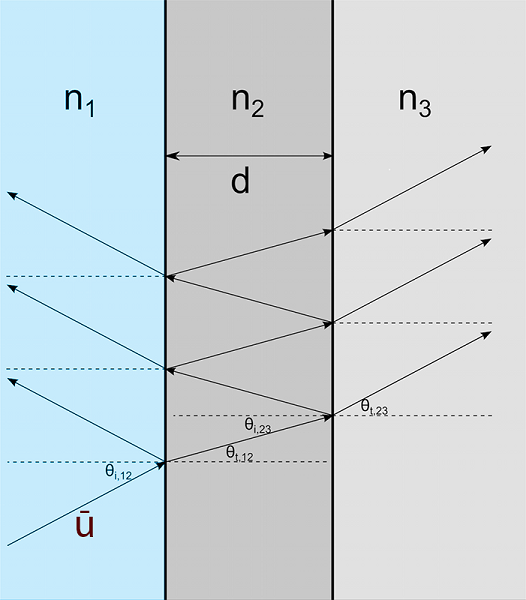Fig 1. Geometry of light propagation in a layered model

Consider a semi-infinite slab of material (base substrate) coated with a layer of some varnish, and assume that all surfaces are perfectly smooth (exhibit no microfacets with a normal different from the macro-surface normal):

1. Light hitting the material with direction u is partially reflected and doesn’t enter the varnish, likewise it is partially refracted and transmitted into the top layer.
2. Ignoring sub-surface scattering, the transmitted portion of the light is then reflected and refracted again at the surface of the substrate.
3. The light refracted at substrate surface (n2 to n3) enters the substrate and might exist at a different point, or is fully absorbed.
4. Light reflected at the substrate surface undergoes reflection and refraction again at the varnish surface. This time refracted energy exits the material, while the reflected energy is goes downwards. Parts of the reflected energy might escape at a different point, be absorbed by the material, or be trapped inside the medium.

The actual amount of energy that undergoes reflection is governed by Fresnel equations and depends only upon the ratio of the (wave-length dependant) refractive-indices of the materials and incident angle. Energy that isn’t reflected is refracted, with the refraction direction is determined by Snell’s Law, which, like the Fresnel equations, depend only upon the ratio of refractive-indices and incident angle.

Energy inside a medium undergoes attenuation, with part of the energy absorbed. The total amount of energy lost before hitting the next layer depends on the path length and attenuation coefficients (which are wave-length dependent) and is governed by the Beer-Lambert Law. If we assume layers of constant thickness the path length is simply $d\times \left|\vec{n}\cdot \vec{v}\right|$, where d is layer thickness, n is the macro-surface normal and v is the light direction inside the medium.

# Simplifications in our model

The BRDF and BTDF computation process, for given incident and outgoing vectors, is a recursive process where we start at the top layer and work our way down to the substrate, i.e. we trace, in real-time, the light path through the layers. To do so with acceptable performance a few assumptions are made:

1. Downwards reflections are ignored: We ignore the energy contribution of light that is reflected off a layer surface, and then at some point reflected again downwards. For this energy to escape the material it must be reflected at least once again, therefore in most cases it doesn’t provide a significant contribution to the BRDF. This allows us to always sample the entire BRDF by tracing through the layers once, and only once.
2. The light incident and exit points are the same: We assume that a layer’s thickness is large compared to a microfacet size, but small enough compared to the size of a shaded fragment so that the distances between the light incident point the (multiple) exit points of the scattered energy are negligible. (That is, we do not attempt to evaluate the BSSDF).

Those simplifications allow us to efficiently sample the BRDF and BTDF in real-time. Weidlich et al.  make the same assumptions, and despite that achieve very good results. However, unlike Weidlich et al. we don’t assume that the incident and exit points at each layer intersect the same microfacet, but that light interacts with all microfacets at each incident and exit point on each layer. This allows us to evaluate the BRDF and BTDF with a single sample.

# Light transport through a microfacet layer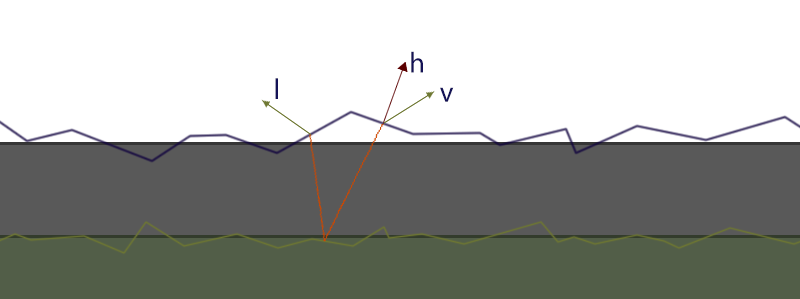Fig 2. Light entering a microfacet slab with incident vector v and exiting with outgoing vector l. The solid slabs represent the layers and the macro-surfaces.

In a microfacet model light interacts in a similar fashion to the smooth scenario, the main difference is that the normals of surface changes for each microfacet. We assume that a microfacet is much smaller than a shaded fragment, therefore incoming light interacts with many microfacets. This means that we need to be able to calculate the total transmission of all involved microfacet, and the average refracted direction.

Transmission through a microfacet surface with roughness $\nu$ and refractive-index ratio $\eta$, such that $\eta = \frac{\eta_2}{\eta_1}$, where $\eta_1$ is the index-of-refraction of the upper medium (the one light is coming from) and $\eta_2$ is the index-of-refraction of the material itself (the one light enters), with given incident direction v:

$$t\left(\vec{v},\nu,\eta\right) = \frac{\int_\Omega P_\nu(\vec{\omega})(1 - F_\eta(\vec{v}\cdot\vec{\omega}))V(\vec{v},\vec{\omega})d\vec{\omega}}{\int_\Omega P_\nu(\vec{\omega})V(\vec{v},\vec{\omega})d\vec{\omega}}$$

Where $P_\nu(\vec{\omega})=D_\nu(\vec{\omega})cos(\theta)$ is the probability distribution function of the microfacet slope, with $D_\nu(\vec{\omega})$ being the NDF for roghness $\nu$, $F_\eta(\vec{v}\cdot\vec{\omega})$ is the fresnel reflection term and

$$V(\vec{v},\vec{\omega})= \begin{cases} 1 &\;\text{if }\vec{v}\cdot\vec{\omega}>0\\ 0 &\;\text{otherwise}\ \end{cases}$$

is the visibility function.

$t\left(\vec{v},\nu,\eta\right)$ calculates the everage fresnel transmission of the microfacets based on their distribution and normalizes over visible microfacets. In spherical coordinates with $\vec{v}=\left(\theta_v, \phi_v\right)$ it becomes:

\begin{aligned} &\int_\Omega } P_\nu(\vec{\omega})(1 - F_\eta(\vec{v}\cdot\vec{\omega}))V(\vec{v},\vec{\omega})d\vec{\omega} = \\ =&\int_0^{\frac{\pi}{2} }\int_0^{2\pi} } D_\nu(\theta,\phi)(1 - F_\eta(\gamma))V(\gamma)\cos\theta \sin\theta d\phi d\theta = \\ =&\frac{1}{2}\int_0^{\frac{\pi}{2} } } \sin\left(2\theta\right) \int_0^{2\pi} } D_\nu(\theta,\phi)(1 - F_\eta(\gamma))V(\gamma)d\phi d\theta\\ &\gamma=arccos(\sin\theta \sin\theta_v + \cos\theta \cos\theta_v \cos\left(\phi-\phi_v\right))\text{ is the arc}\\ &\text{angle between }(\theta_v, \phi_v)\text{ and }(\theta, \phi) \end{aligned}

The last equality uses the trigonometric identity $\sin\left(2x\right)=2\cos x\sin x$.
Unfortunately neither integral has a closed form solution for common NDFs but are very suitable for numeric integration thanks to very smooth integrands. Smith et al.  arrive at the same conclusion, though they ignore the required normalization of the total Fresnel transmission based on total visible microfacets.

Calculating the average refraction direction is done in a similar way:

$$r\left(\vec{v}, \vec{n},\nu,\eta\right) = \int_\Omega }P_\nu(\vec{\omega})\mathrm{O}(\mathrm{refract}(\vec{v},\vec{\omega},\eta),\vec{n})d\vec{\omega}$$

Where

$$\mathrm{refract}(\vec{v},\vec{n},\eta)= \begin{cases} -\frac{\vec{v}}{\eta}+\vec{n}\left(\frac{\vec{n}\cdot\vec{v}}{\eta} - \sqrt{1 - \frac{1 - \left(\vec{n}\cdot\vec{v}\right)^2}{\eta^2}}\right) &\;\text{if }1 - \left(\vec{n}\cdot\vec{v}\right)^2\le\eta^2\\ \vec{0} &\;\text{otherwise}\ \end{cases}$$ computes the refracted vector and $$\mathrm{O}(\vec{v},\vec{n})= \begin{cases} \vec{v} &\;\text{if }\vec{v}\cdot\vec{n} < 0 \\ \vec{0} &\;\text{otherwise}\ \end{cases}$$

discards vectors which refract outside the macro-surface. The resulting vector from the integral is unnormalized and gives the average refraction direction. The result can also be the zero vector which means all the visible microfacets refract outside the macro-surface or undergo total-internal-reflection. Note that again we ignore multi scattering.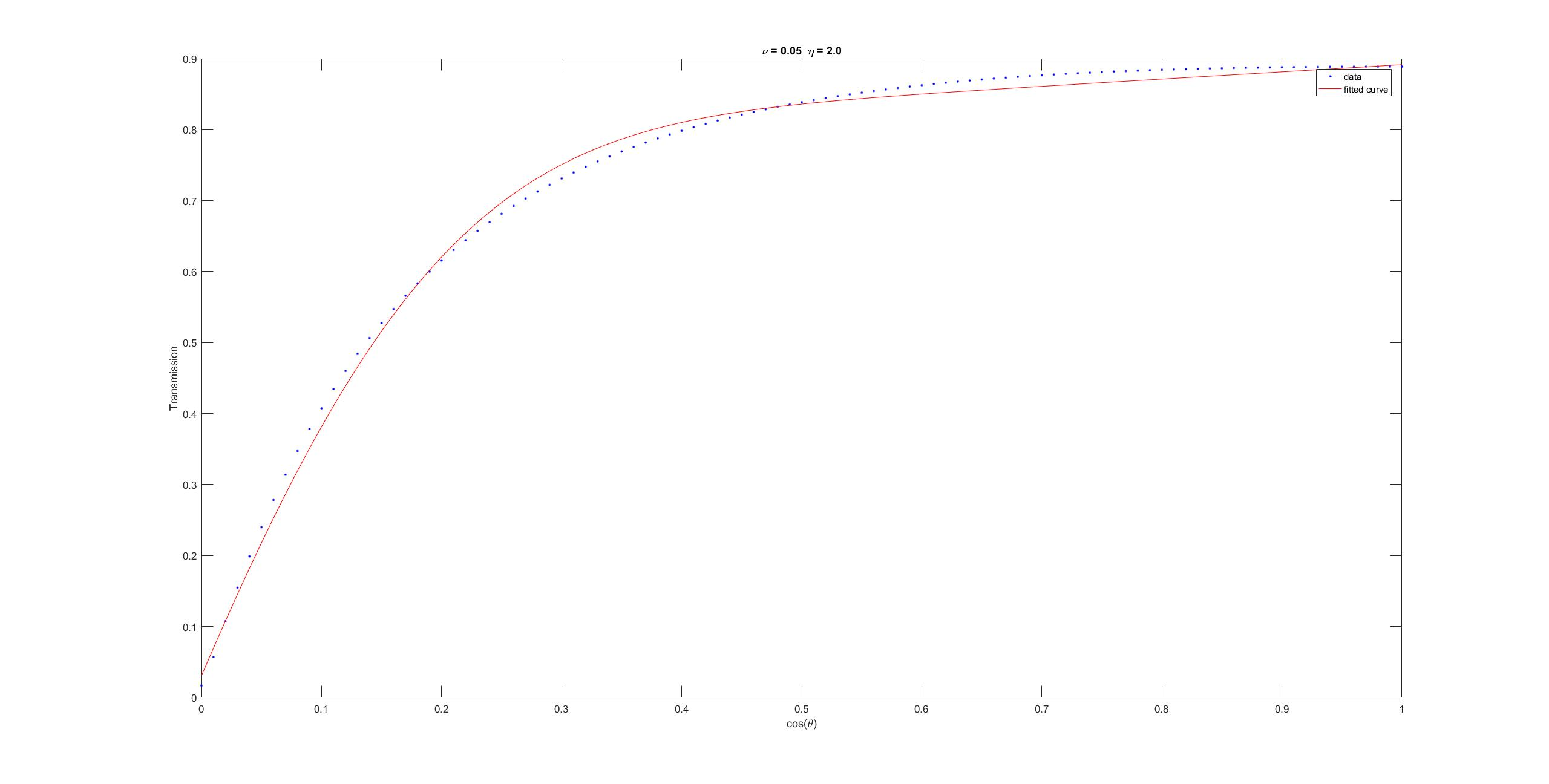Roughness: 0.05 - IOR ratio: 2.0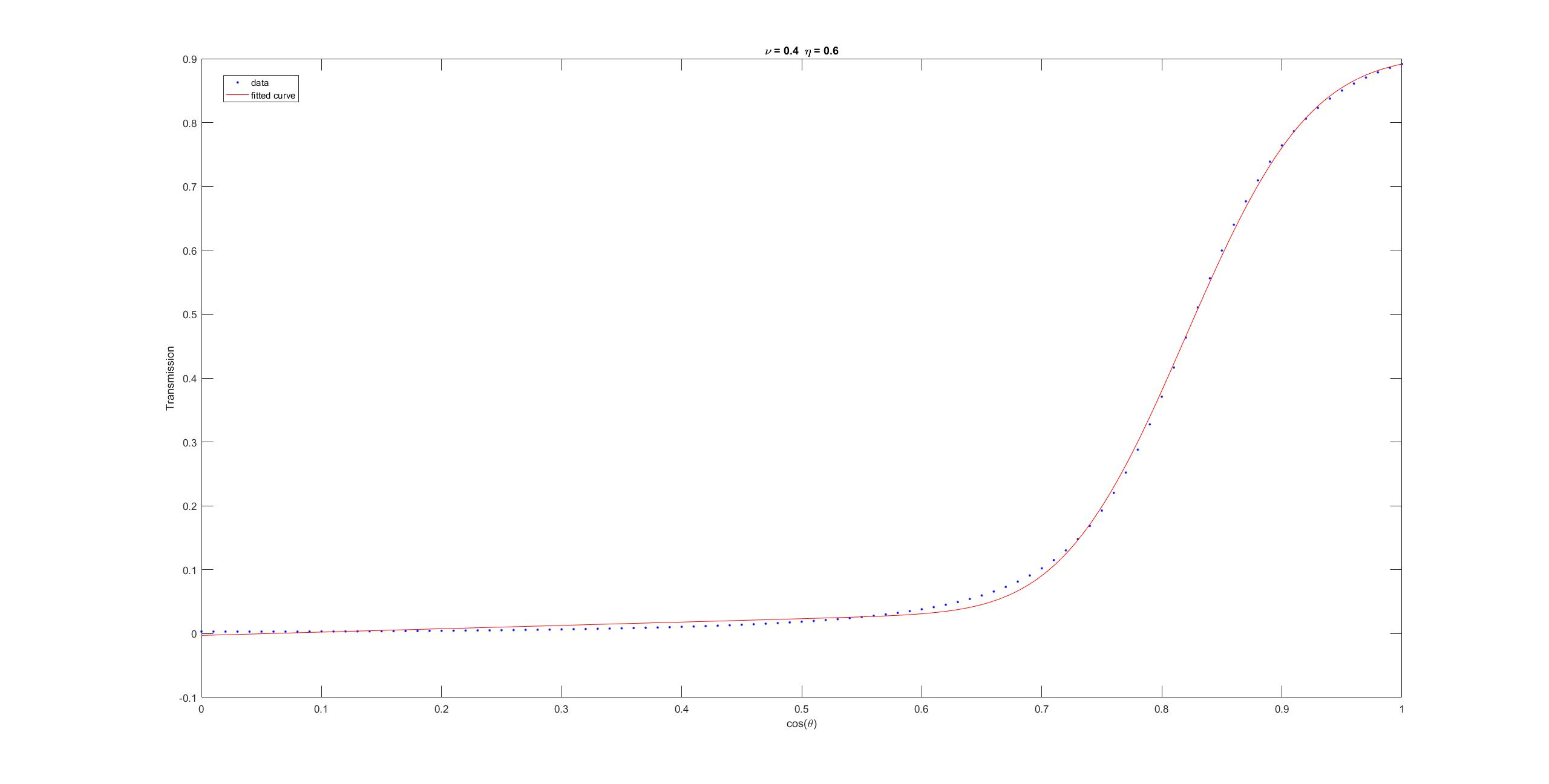Roughness: 0.4 - IOR ratio: 0.6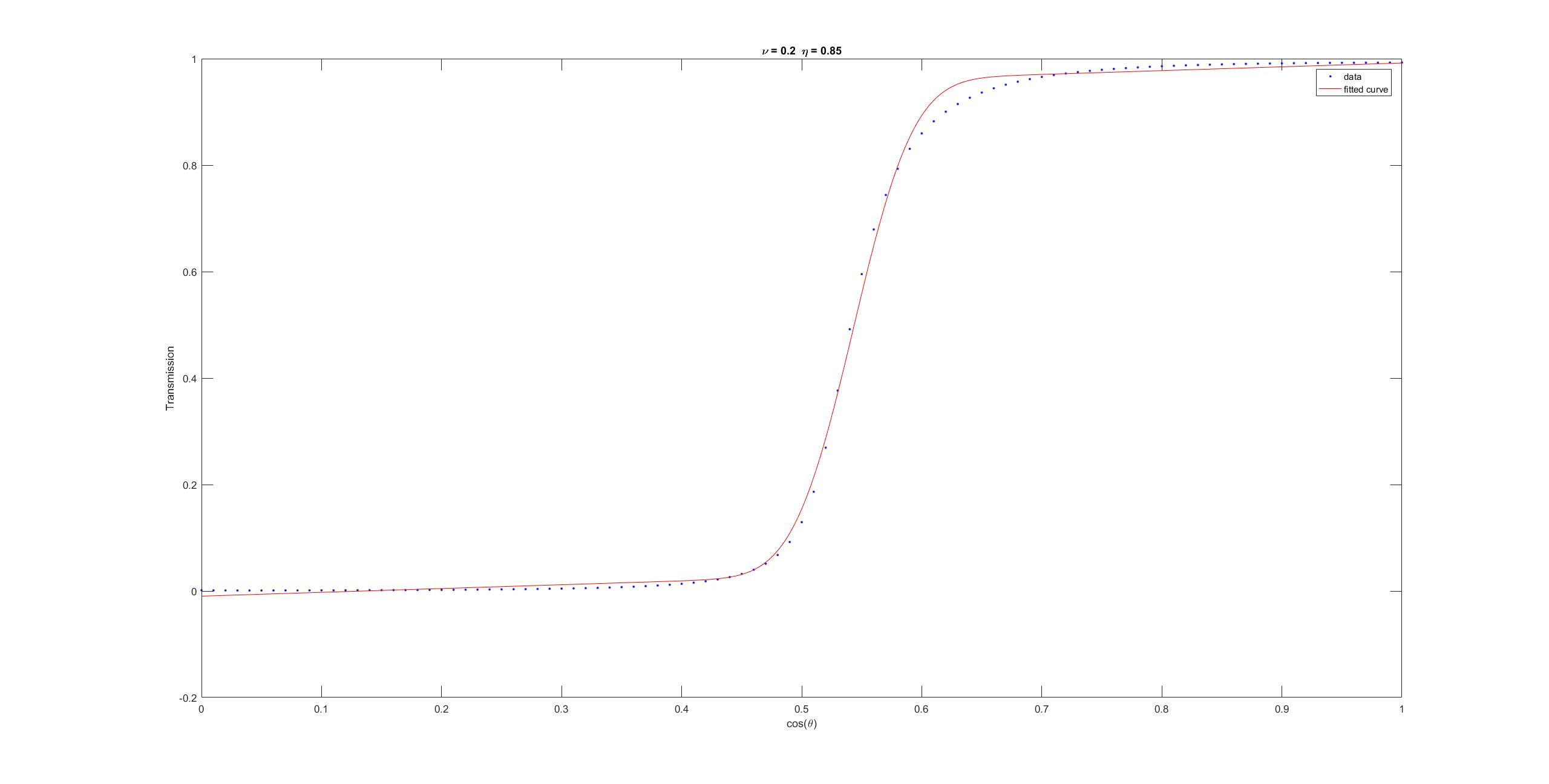Roughness: 0.2 - IOR ratio: 0.85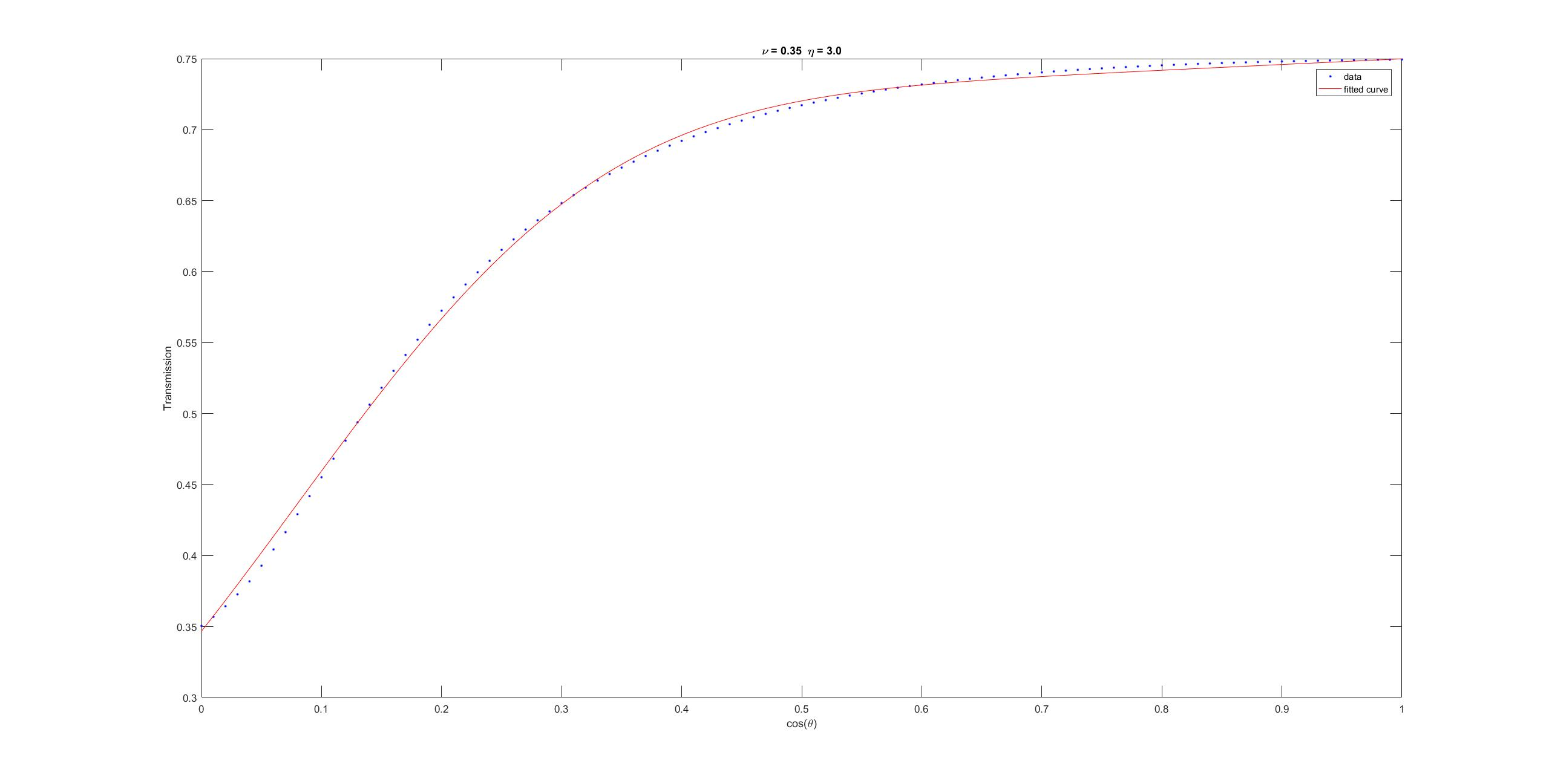Roughness: 0.35 - IOR ratio: 3.0
Fig 3. Plots of fitted curve of transmission as function of cosine of incident angle for various roughness and refractive-index ratio values

Like the transmission term, this has no closed form solution. Our approach is to generate 2D LUTs indexed by roughness and refractive-index ratio pairs ($\nu$, $\eta$). For each pair ($\nu$, $\eta$) values for $t\left(\vec{v},\nu,\eta\right)$ and $r\left(\vec{v}, \vec{n},\nu,\eta\right)$ are numerically integrated for $\theta\in\left[0,\frac{\pi}{2}\right]$ and a curve fitting approximation is generated, the coefficients for the curve fitting function are stored in the LUT. The curve fitting function that works well is a double exponent $a_1 e^{a_2 x} + b_1 e^{b_2 x}$ for refraction direction. For transmission, due to the curve shape resembling a Sigmoidal, the function $m((\textrm{erf}(ax - b) + 1) + cx) + d$ provided good fits. The error function can be approximated analytically with a 3-degree polynomial and an exponent .

# Diffuse

Each layer exhibits a diffuse component, our model assumes that it is the result of part of the attenuated light undergoing subsurface scattering and emerging fully diffused. Therefore the diffuse color is $t_i t_o((1-k)\odot c)$, where k is per-component attenuation through the layer, c is the albedo, $t_i\text{, }t_o$ are the inwards and outwards (incident and outgoing from inside the layer) transmission terms respectively and $\odot$ is component-wise multiplication.
We use the Lambert diffuse BRDF (simply $\frac{1}{\pi}$), any other BRDF can be used. As noted by Disney , the Lambert BRDF makes rough surfaces look dark around the edges, and adding the Fresnel terms makes it worse. While that is true, it should be remedied by adding a physically plausible multiple-scaterring component to the model.

# Evaluating a multi-layered BRDF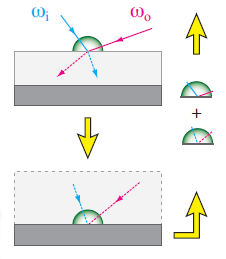Similar to Weidlich et al.  we path trace from top to bottom of the layer stack, updating the incident and outgoing vectors at each layer.

Given incident vector v, outgoing vector l, layer stack with roughness value $\nu$ and refractive-index ratio $\eta$ per layer we evaluate the BRDF by ray tracing through the stack, evaluating the BRDF at each layer and summing up.
We use a couple of variables: Accumulated attenuation variable q (initialized to 1), and accumulated outgoing radiance variable r (initialized to 0).

1. Compute inwards and outwards transmission terms $t_i\text{, }t_o$.
2. Compute refracted vectors u, o from $\nu$, l respectively.
3. Compute the total path length inside the layer:

$l = d\left(\frac{1}{\vec{n}\cdot\vec{u}} + \frac{1}{\vec{n}\cdot\vec{o}}\right)$ where d is the layer thickness and n is the macro-surface normal.

4. Compute the attenuation inside the layer:

$k = e^{a(\lambda)l}$ where $a(\lambda)$ is the wave-length dependent attenuation coefficient.

5. Evaluate the layer BRDF using v and l as incident and outgoing vectors, and diffuse color as described above. Multiply the layer BRDF by the attenuation q and add to r.

6. Set v and l to u and o respectively.

7. Multiply q by layer attenuation:

$k t_i t_o G$, where G is the microfacet shadowing-masking term.

8. Continue to next layer.

# Dielectrics and conductors

Metals conduct electricity, incoming light stimulates the material electrons resulting in specular reflection (re-emission), which happens mostly at the surface. Therefore, unlike dielectrics, conductors have no internal scattering and no true diffuse contribution to the BRDF. Another important conclusion is that the specular highlight color of conductors is the color of the material and not light color, like most dielectrics.

# Conclusion and future work

The multi-layered model presented allows us to intuitively construct multi-layered microfacet materials and evaluate their BRDF in real-time using a physically-based approach with good results. Future work will involve multiple-scattering and subsurface-scattering based around this model as those scatterings constitute a noticeable portion of the BRDF energy of rough materials at grazing angles.

A note about anisotropy: Anisotropic NDFs take 2 roughness values which would require 3D LUTs. A solution would be to find analytic approximation to the aforementioned transmission and refraction integrals or to find a fit to 2D/3D data. This is desired anyway to either reduce the LUTs dimension (greatly reducing precomputation time) or avoid LUTs altogether.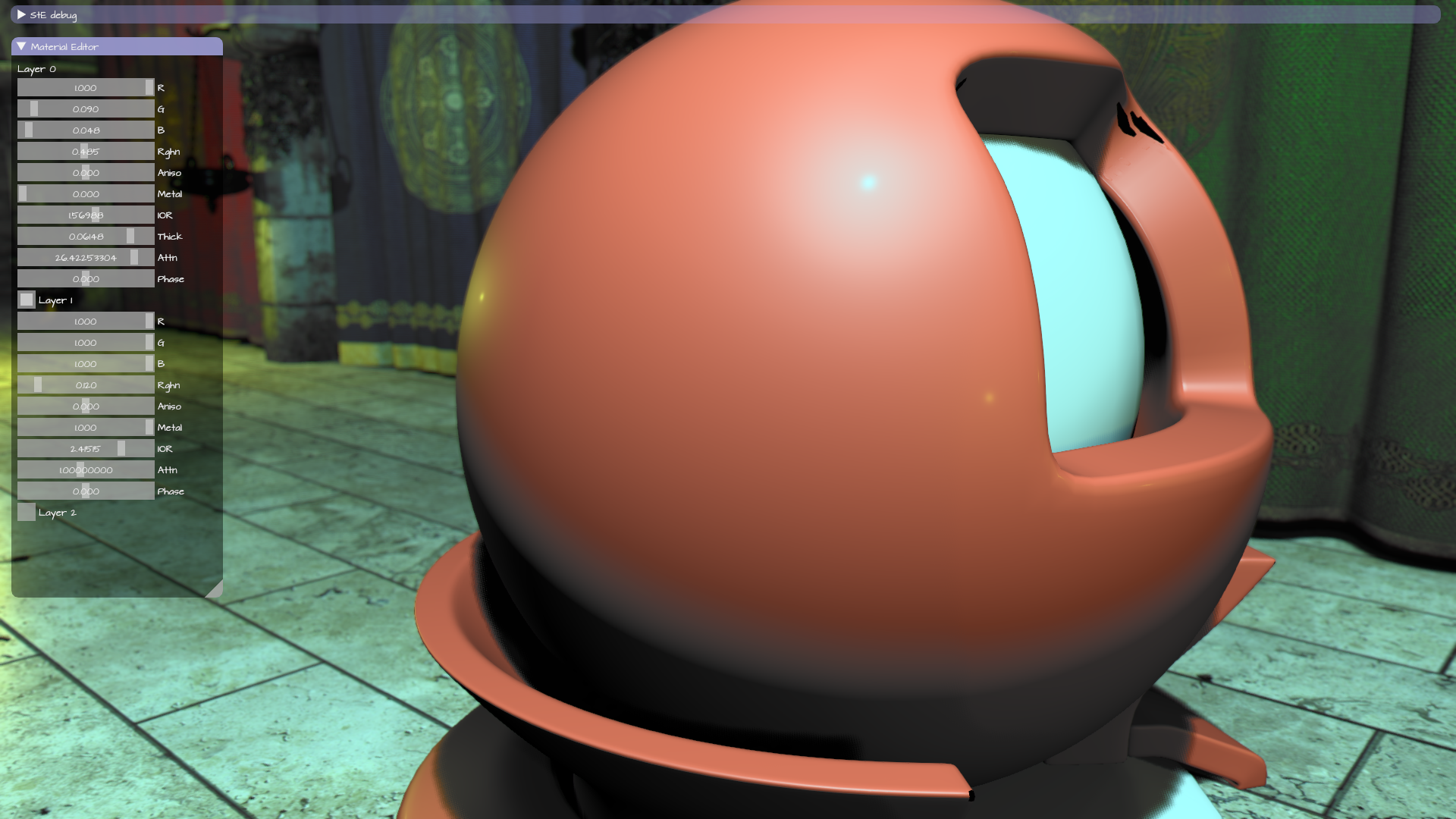Frosted metal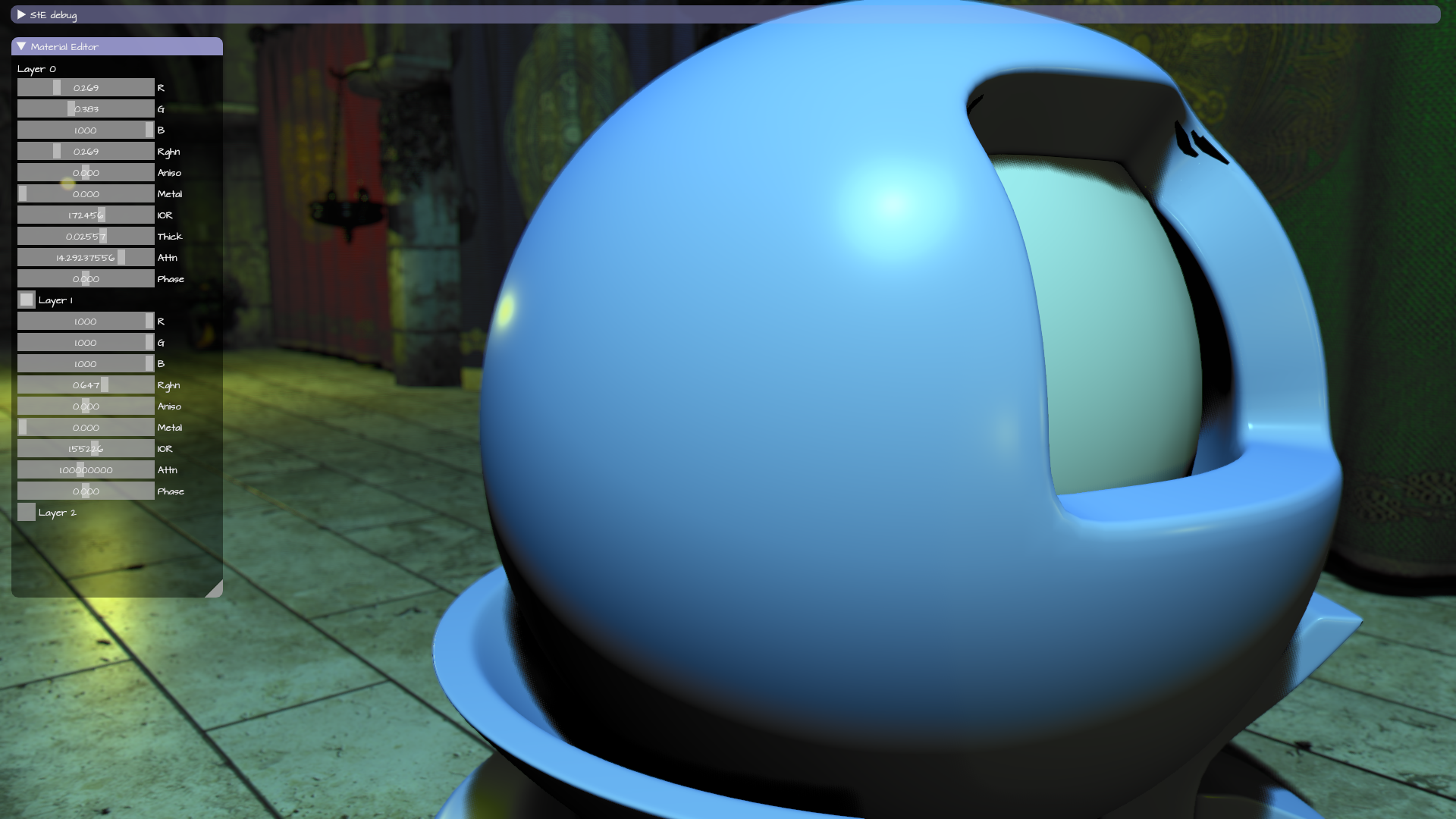Patina: Rough metal base with rough paint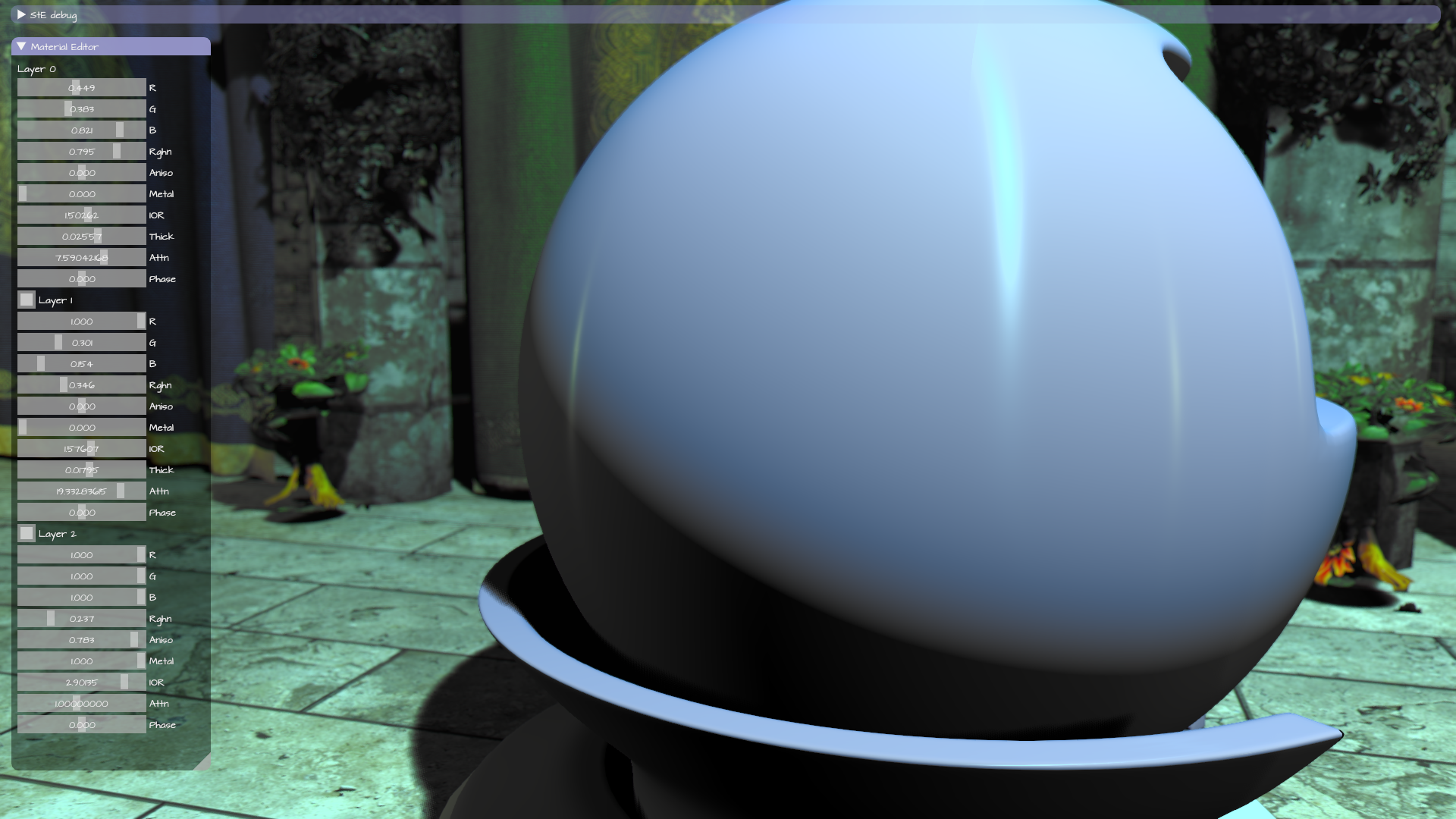Brushed metal with 2 layers of differently colored paints

# Appendix: Code and LUTs

GLSL code sample for evaluating a multi-layered BRDF is available at https://github.com/ssteinberg/ste/blob/master/Simulation/src/ste/framework_graphics/material/shaders/material_evaluate.glsl

Fitting code for the LUTs is available at https://github.com/ssteinberg/ste/tree/master/Simulation/Supplemental

LUTs:

GLSL code sample for querying the LUTs

 Torrance, Kenneth E. and Sparrow, Ephraim M., Theory for Off-Specular Reflection From Roughened Surfaces, Journal of the Optical Society of America, v.57 pp.1105-1114, September 1967.

 Andrea Weidlich and Alexander Wilkie. Arbitrarily layered micro-facet surfaces. In Proceedings of the 5th international conference on Computer graphics and interactive techniques in Australia and Southeast Asia (GRAPHITE ’07). ACM, New York, NY, USA, 171-178, 2007.

 Bruce Walter, Stephen R. Marschner, Hongsong Li, and Kenneth E. Torrance. Microfacet models for refraction through rough surfaces. In Proceedings of the 18th Eurographics conference on Rendering Techniques (EGSR’07), Jan Kautz and Sumanta Pattanaik (Eds.). Eurographics Association, Aire-la-Ville, Switzerland, Switzerland, 195-206. 2007.

 Brent Burley, Physically-Based Shading at Disney. _https://disney-animation.s3.amazonaws.com/library/s2012_pbs_disney_brdf_notes_v2.pdf_

 William A. P. Smith and Edwin R. Hancock. Specular and diffuse reflectance in microfacet models. In Proceedings of the 16th IEEE international conference on Image processing (ICIP’09), Magdy Bayoumi (Ed.). IEEE Press, Piscataway, NJ, USA, 3737-3740, 2009.

 Eric Heitz, Understanding the Masking-Shadowing Function in Microfacet-Based BRDFs, Journal of Computer Graphics Techniques (JCGT), vol. 3, no. 2, 48-107, 2014

 How Is The NDF Really Defined?, _http://www.reedbeta.com/blog/hows-the-ndf-really-defined/, 2013_

 Specular BRDF Reference, _http://graphicrants.blogspot.co.il/2013/08/specular-brdf-reference.html, 2013_

 Abramowitz and Stegun: Handbook of Mathematical Functions: Error Function and Fresnel Integrals, _http://people.math.sfu.ca/~cbm/aands/page_299.htm_